﻿ 蒸发换热器与变压器连接位置对流动换热的影响 The Influence of Connection Position between Evaporation Heat Exchanger and Transformer on Flow and Heat Exchange

Sustainable Energy
Vol.08 No.02(2018), Article ID:24832,9 pages
10.12677/SE.2018.82003

The Influence of Connection Position between Evaporation Heat Exchanger and Transformer on Flow and Heat Exchange

Tingting Liu, Yongguang Li

School of Energy and Mechanical Engineering, Shanghai University of Electric Power, Shanghai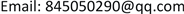Received: Mar. 20th, 2018; accepted: May 4th, 2018; published: May 11th, 2018ABSTRACT

The fluent software was used to simulate and analyze the underground transformer and evaporative heat exchanger model, and the characteristics of the oil flow between the transformer and the evaporative heat exchanger and the movement of the surrounding air were obtained. Comparing and analyzing the flow field and temperature field of evaporative heat exchangers at different connection positions, it is concluded that the heat transfer conditions of the evaporative heat exchanger are better and the flow velocity of the fluid at the connection position is the fastest.

Keywords:Underground Transformer, Evaporative Heat Exchanger, Simulation, Heat Dissipation1. 前言

2. 模型的建立

2.1. 物理模型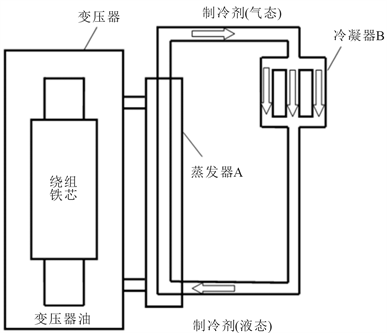Figure 1. Evaporative cooling schematic

1) 考虑到模拟中油的流动主要在变压器铁芯绕组外部与蒸发器之间，故暂忽略铁芯和高、低压绕组内部的导热问题。

2) 由于变压器流场呈对称分布，故取此变压器流场的1/2进行数值模拟，计算模型如图2所示。

3) 最外部的正方体模拟环境边界，尺寸为4000 mm × 4000 mm × 4000 mm。

2.2. 控制方程

2.2.1. 连续方程

$\frac{\partial \rho }{\partial t}+\frac{\partial \left(\rho u\right)}{\partial x}+\frac{\partial \left(\rho v\right)}{\partial y}+\frac{\partial \left(\rho w\right)}{\partial z}=0$ (1)

2.2.2. 动量守恒方程

$\frac{\partial \left(\rho u\right)}{\partial t}+div\left(\rho uU\right)=-\frac{\partial p}{\partial x}+\frac{\partial {\tau }_{xx}}{\partial x}+\frac{\partial {\tau }_{yx}}{\partial y}+\frac{\partial {\tau }_{zx}}{\partial z}+{F}_{x}$ (2)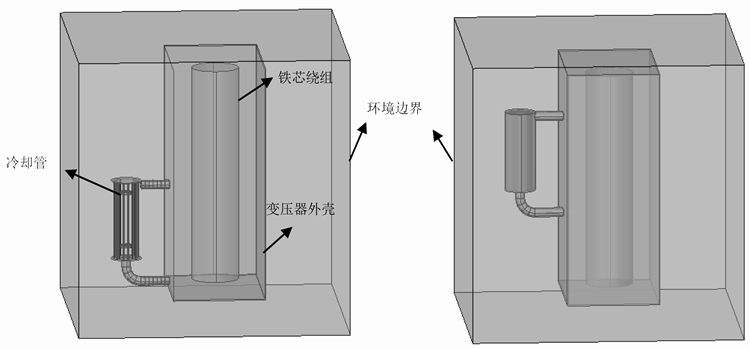Figure 2. Comparison of models of evaporative heat exchangers in different locations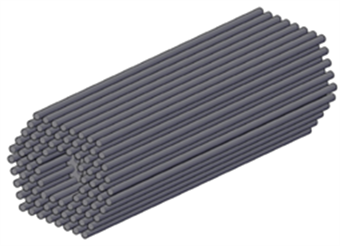Figure 3. Cooling pipe arrangementFigure 4. Cross section of baffle and cooling tube

$\frac{\partial \left(\rho v\right)}{\partial t}+div\left(\rho vU\right)=-\frac{\partial p}{\partial x}+\frac{\partial {\tau }_{xy}}{\partial x}+\frac{\partial {\tau }_{yy}}{\partial y}+\frac{\partial {\tau }_{zy}}{\partial z}+{F}_{y}$ (3)

$\frac{\partial \left(\rho w\right)}{\partial t}+div\left(\rho wU\right)=-\frac{\partial p}{\partial x}+\frac{\partial {\tau }_{xz}}{\partial x}+\frac{\partial {\tau }_{yz}}{\partial y}+\frac{\partial {\tau }_{zz}}{\partial z}+{F}_{z}$ (4)

2.2.3. 能量守恒方程

$\frac{\partial \left(\rho T\right)}{\partial t}+div\left(\rho UT\right)=div\left(\frac{\lambda }{{c}_{p}}gradT\right)+{S}_{T}$ (5)

$\rho ={\rho }_{0}\left[1-\beta \left(T-{T}_{0}\right)\right]$ (6)

${\rho }_{0}$ 为流体密度，kg/m3；T0为环境温度，K； $\beta$ ——热膨胀系数，1/K；

2.3. 网格划分Table 1. Physical properties of transformer insulation oil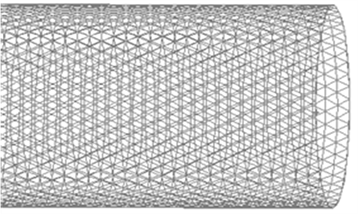Figure 5. Cooling pipe local grid

2.4. 边界条件与求解器设置

3. 数值模拟的结果

3.1. 流场情况

3.2. 速度矢量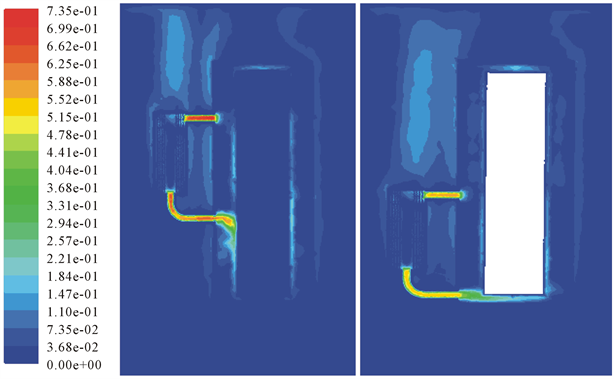Figure 6. Flow field distribution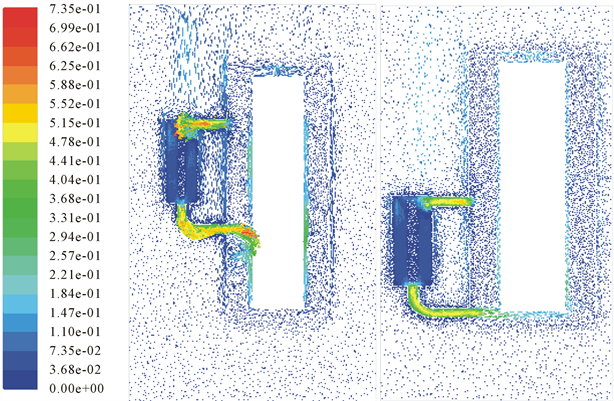Figure 7. Speed vector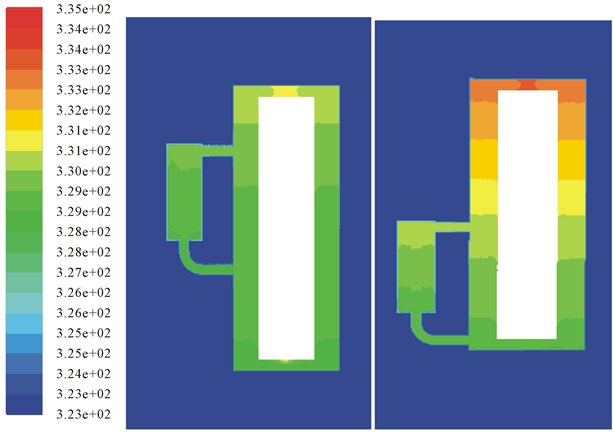Figure 8. Temperature distribution

3.3. 温度分布

4. 结论

1) 本文通过模拟变压器油在地下变压器与蒸发换热器之间的流动，得到了其流场和温度场，这对地下变压器的冷却提供了一个新的改进思路和优化方案。

2) 顶层油温较高，速度也大，且在油箱顶部形成了旋涡，增强了换热。自然对流时在变压器和蒸发换热器的接口处以及铁芯绕组附近油流动的速度最快。

3) 通过对比不同位置蒸发换热器模型的流动和传热情况，发现蒸发换热器和变压器连接位置对变压器散热有一定的影响，相同工况下，蒸发换热器位置高的换热效果更好。

The Influence of Connection Position between Evaporation Heat Exchanger and Transformer on Flow and Heat Exchange[J]. 可持续能源, 2018, 08(02): 23-31. https://doi.org/10.12677/SE.2018.82003

1. 1. 姜益民. 城市电力变压器的发展趋势[J]. 变压器, 2005, 42(8): 1-4.

2. 2. 何永秀, 朱茳, 罗涛, 等. 城市电网规划自然灾害风险评价研究[J]. 电工技术学报, 2011, 26(12): 205-210.

3. 3. 卞辉, 姜益民, 袁聪波, 等. 强油油冷式冷却方式在城市地下变电站中的应用[J]. 变压器, 2011, 48(8): 43-47.

4. 4. 于海波. 关于地下变压器冷却系统的分析[J]. 通信电源技术, 2011, 28(6): 80-81.

5. 5. 成展鹏, 胡佐. 一起主变强油风冷回路不正常启动的分析[J]. 电力系统保护与控制, 2009, 37(5): 93-95.

6. 6. 祝丽花, 杨庆新, 闫荣, 等. 考虑磁致伸缩效应电力变压器振动噪声的研究[J]. 电工技术学报, 2013, 28(4): 1-6.

7. 7. 哈恩E, 格里占尔U. 沸腾换热[M]. 王兴国, 华诚生, 译. 北京: 国防工业出版社, 1988.